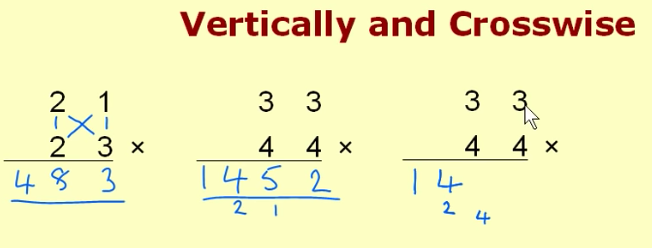```           3 = 1*3   Rightmost digit is product of two rightmost
8 = 2*3 + 2*1  Tens digit is sum of two products (tens-column * ones-column)
4 = 2*2           Hundreds digit is product of tens digits.
NOTE: No carrys!   (Carrys will go under next column left.)

3*4 = 12:     // 2 in ones-column; carry 1 underneath, to tens-column.
3*4 + 3*4 = 24:  // add carried 1 for 5, with 2 carried underneath to 100s col.
3*4 = 12:          // add carried 2 for 4 in hundreds-column;  carried 1 goes in 1000s-col.

Same can be done Right-To-Left.
(carries go underneath next column to right!)

3*4 is 12;       // 1 in 1000s column, 2 underneath 100s col.
3*4 + 3*4 = 24;    // 2+carry=4, 4 under tens col.
3*4 = 12;            // adding carried 4 puts 5 in tens column, 2 into ones-column.

http://mathlearners.com/

Vedic Math
*
Example
*
Fast Mul.
*
Multiply
```